Courses

# True/False of Electrostatics, Past year Questions JEE Advance, Class 12, Physics JEE Notes | EduRev

## JEE : True/False of Electrostatics, Past year Questions JEE Advance, Class 12, Physics JEE Notes | EduRev

The document True/False of Electrostatics, Past year Questions JEE Advance, Class 12, Physics JEE Notes | EduRev is a part of the JEE Course Class 12 Physics 35 Years JEE Mains &Advance Past year Paper.
All you need of JEE at this link: JEE

True/False

Q.1. The work done in carrying a point charge from one point to another in an electrostatic field depends on the path along which the point charge is carried.     (1981- 2 Marks)

Ans. F

Solution. Electrostatic force is conservative in nature, therefore work done is path independent.

Q.2. Two identical metallic spheres of exactly equal masses are taken. One is given a positive  charge Q coulombs and the other an equal negative charge. Their  masses after charging are different.    (1983 - 2 Marks)

Ans. T

Solution. The metallic sphere which gets negatively charged gains electrons and hence its mass increases.

The metallic sphere which gets positively charged loses electrons and hence its mass decreases.

Q.3. A small metal ball is suspended in a uniform electric field with the help of an insulated thread. If high energy X-ray beam falls on the ball, the ball will be deflected in the direction of the field. (1983 - 2 Marks)

Ans. T

Solution. When high energy X-ray beam falls, it will knock out electrons from the small metal ball making it positively charged.

Therefore the ball will be deflected in the direction of electric field.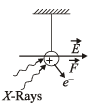Q.4. Two protons A and B are placed in between the two plates of a parallel plate capacitor charged to a potential difference V as shown in the figure. The forces on the two protons are identical.     (1986 - 3 Marks)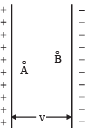Ans. T

Solution. The electric field produced between th e parallel plate capacitor is uniform. The force acting on charged particle placed in an electric field is given by F = qE.

In the case of two protons, q and E are equal and hence force will be equal.

Q.5. A ring of radius R carries a uniformly distributed charge + Q.

A point charge – q is placed on the axis of the ring at a distance 2R from the centre of the ring and released from rest. The particle executes a simple harmonic motion along the axis of the ring.     (1988 - 2 Marks)

Ans. F

Solution. KEY CONCEPT : Force on charge (– q) due to small charge dq situated at length dℓ is given by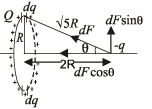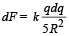Resolving this force into two parts dFcos θ and dFsin θ as shown in figure.

If we take another diametrically opposite length d, the charge on it being dq. Then the force on charge (– q) by this small charge dq will be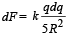Again resolving this force, we find dFsin q components of the two forces cancel out and dFcos q component adds up.

∴ The total force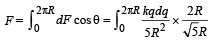Charge on length 2πR = Q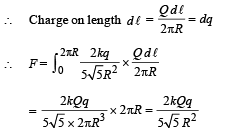This is not an equation of simple harmonic motion.

Q.6. An electric line of forces in the x – y plane is given by the equation x2 + y2 = 1. A particle with unit positive charge, initially at rest at the point x = 1, y = 0 in the x – y plane, will move along the circular line of force.     (1988 - 2 Marks)

Ans. F

Solution. For a particle to move in circular motion, we need a centripetal force which is not available.

The statement is false.

Offer running on EduRev: Apply code STAYHOME200 to get INR 200 off on our premium plan EduRev Infinity!

42 docs|19 tests

,

,

,

,

,

,

,

,

,

,

,

,

,

,

,

,

,

,

,

,

,

,

,

,

,

,

,

,

,

,

;